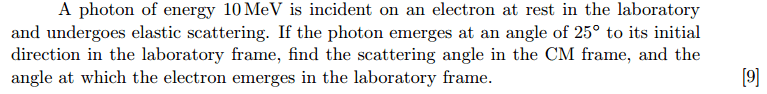# Compton Scattering Angle - CM FRAME

unscientific

## Homework Statement

Given incoming photon has energy 10Mev and scatters at angle 25 degrees, find the scattering angle in CM frame.## The Attempt at a Solution

[/B]
In lab frame, let the energy of incoming photon be ##E##. Total 4-vector would be ##P + Q = (\frac{E}{c},\frac{E}{c}) + (mc,0)##.

Using compton scattering formulas, I solved the scattering angle of electron ##\alpha = 35 ^o##, energy of scatted electron ##E_e = 4.02 ~MeV##, energy of scattered photon ##E' = 5.47~MeV##.

Having solved this completely in the lab frame before/after scattering, how do I proceed in CM-frame?

In CM frame, total momentum is ##0##, so 4-vector would be ##P_{cm} + Q_{cm} = (\frac{E_{p,cm}+E_{e,cm}}{c},0)##.

Even when I assume that in CM frame they are both scattered along the same line it doesn't help.

Staff Emeritus